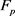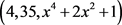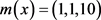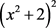# 有限域上的不可约多项式RSA体制Irreducible Polynomials about RSA Type Public Key Cryptosystem over Finite Fields

• 全文下载: PDF(460KB)    PP.1411-1417   DOI: 10.12677/AAM.2018.711165
• 下载量: 460  浏览量: 4,109

Finite field is one of the most basic mathematical tools of computer science and digital communi-cation field, as well as one of the important branches of modern mathematics. The general theory of finite field mainly starts from the Gauss and Galois, but in recent decades, with the development of discrete mathematics, many mathematicians engaged in applied research and paid attention to the research and application of theory of limited. At the same time, the polynomial theory, especially the properties of irreducible polynomials to analyze various performances of pseudorandom sequence, has a special performance, so the studies of the irreducible polynomials over finite field have been widespreadly concerned in mathematical, coding and cryptology research. This paper found that the PK-RSA simulated security is higher by comparing the irreducible polynomials over finite field to the system of three RSAs.

1. 引言

2. 基于有限域上不可约多项式的RSA的模拟一

2.1. 密钥生成

1) 假设p和q都是大素数，且满足 $p ，选择 ${F}_{p}$ 上的一个首项系数为1的m次多项式为 $g\left(x\right)$ ，使其在 ${F}_{p}$ 上满足分解式 $g\left(x\right)={g}_{1}^{\left(1\right)}\left(x\right){g}_{2}^{\left(1\right)}\left(x\right)\cdots {g}_{{k}_{1}}^{\left(1\right)}\left(x\right)$ ，其中 ${g}_{i}^{\left(1\right)}\left(x\right)$${F}_{p}$ 上的 ${m}_{i}^{\left(1\right)}$ 次不可约多项式 $\left(i=1,2,\cdots ,{k}_{1}\right)$ ，同时 $g\left(x\right)$${F}_{q}$ 上满足分解式：

$g\left(x\right)={g}_{1}^{\left(2\right)}\left(x\right){g}_{2}^{\left(2\right)}\left(x\right)\cdots {g}_{{k}_{2}}^{\left(2\right)}\left(x\right)$${g}_{i}^{\left(2\right)}\left(x\right)$${F}_{q}$ 上的 ${m}_{i}^{\left(2\right)}$ 次不可约多项式 $\left(i=1,2,\cdots ,{k}_{2}\right)$

2) 根据欧拉函数的通式易得：

${\varphi }_{p}\left(g\left(x\right)\right)={p}^{m}\underset{i=1}{\overset{{k}_{1}}{\prod }}\left(1-\frac{1}{{p}^{{m}_{i}^{\left(1\right)}}}\right)$${\varphi }_{q}\left(g\left(x\right)\right)={q}^{m}\underset{i=1}{\overset{{k}_{2}}{\prod }}\left(1-\frac{1}{{p}^{{m}_{i}^{\left(2\right)}}}\right)$

3) 根据Euclid算法： ${e}_{1}{d}_{1}\equiv 1\mathrm{mod}\left({\varphi }_{p}\left(g\left(x\right)\right)\right),1<{e}_{1},{d}_{1}<{\varphi }_{p}\left(g\left(x\right)\right)$ ，得：

${e}_{2}{d}_{2}\equiv 1\mathrm{mod}\left({\varphi }_{q}\left(g\left(x\right)\right)\right),1<{e}_{2},{d}_{2}<{\varphi }_{q}\left(g\left(x\right)\right)$

4) 可计算得其公钥为： $\left\{{e}_{1},{e}_{2},g\left(x\right),r\right\}$ ，私钥为： $\left\{{d}_{1},{d}_{2},p,q,{\varphi }_{p}\left(g\left(x\right)\right),{\varphi }_{q}\left(g\left(x\right)\right)\right\}$

2.2. 加密算法

${〈{\left({a}_{m-1}{x}^{m-1}+{a}_{m-2}{x}^{m-2}+\cdots +{a}_{1}+{a}_{0}\right)}^{{e}_{1}}〉}_{g\left(x\right)}={b}_{m-1}^{\left(1\right)}{x}^{m-1}+{b}_{m-2}^{\left(1\right)}{x}^{m-2}+\cdots +{b}_{1}^{\left(1\right)}x+{b}_{0}^{\left(1\right)}$

${〈{\left({a}_{m-1}{x}^{m-1}+{a}_{m-2}{x}^{m-2}+\cdots +{a}_{1}x+{a}_{0}\right)}^{{e}_{2}}〉}_{g\left(x\right)}={b}_{m-1}^{\left(2\right)}{x}^{m-1}+{b}_{m-2}^{\left(2\right)}{x}^{m-2}+\cdots +{b}_{1}^{\left(2\right)}x+{b}_{0}^{\left(2\right)}$

$\left({b}_{m-1}^{\left(1\right)},{b}_{m-2}^{\left(1\right)},\cdots ,{b}_{1}^{\left(1\right)},{b}_{0}^{\left(1\right)};{b}_{m-1}^{\left(2\right)},{b}_{m-2}^{\left(2\right)},\cdots ,{b}_{1}^{\left(2\right)},{b}_{0}^{\left(2\right)}\right)\in {Z}_{r}^{2m}$ 作为密文。

2.3. 解密算法

1) 已知 $\left({b}_{m-1},{b}_{m-2},\cdots ,{b}_{1},{b}_{0}\right)\in {Z}_{r}^{m}$ ，可得：

${c}_{j}^{\left(1\right)}={〈{b}_{j}〉}_{p},{c}_{j}^{\left(2\right)}={〈{b}_{j}〉}_{q},j=0,1,2,\cdots ,m-1$

2) 在 ${F}_{p}$${F}_{q}$ 上分别计算：

${〈{\left({c}_{m-1}^{\left(1\right)}{x}^{m-1}+{c}_{m-2}^{\left(1\right)}{x}^{m-2}+\cdots +{c}_{1}^{\left(1\right)}x+{c}_{0}^{\left(1\right)}\right)}^{{d}_{1}}〉}_{g\left(x\right)}={a}_{m-1}^{\left(1\right)}{x}^{m-1}+{a}_{m-2}^{\left(1\right)}{x}^{m-2}+\cdots +{a}_{1}^{\left(1\right)}x+{a}_{0}^{\left(1\right)}$

${〈{\left({c}_{m-1}^{\left(1\right)}{x}^{m-1}+{c}_{m-2}^{\left(1\right)}{x}^{m-2}+\cdots +{c}_{1}^{\left(2\right)}x+{c}_{0}^{\left(2\right)}\right)}^{{d}_{2}}〉}_{g\left(x\right)}={a}_{m-1}^{\left(2\right)}{x}^{m-1}+{a}_{m-2}^{\left(1\right)}{x}^{m-2}+\cdots +{a}_{1}^{\left(2\right)}x+{a}_{0}^{\left(2\right)}$

3) 解同余方程组：

$\left\{\begin{array}{c}{a}_{j}\equiv {a}_{j}^{\left(1\right)}\mathrm{mod}p\\ {a}_{j}\equiv {a}_{j}^{\left(2\right)}\mathrm{mod}q\end{array}\left(j=0,1,2,\cdots ,m-1\right)$

2.4. 算法分析

3. 基于有限域上不可约多项式的RSA的模拟二

3.1. 密钥生成

1) 假设p和q都是大素数，并且满足 $p ，选择 ${F}_{p}$ 上的一个首项系数是1的m次多项式是 $g\left(x\right)$ ，使得它在 ${F}_{p}$ 上满足分解式 $g\left(x\right)={g}_{1}^{\left(1\right)}\left(x\right){g}_{2}^{\left(1\right)}\left(x\right)\cdots {g}_{{k}_{1}}^{\left(1\right)}\left(x\right)$ ，其中的 ${g}_{i}^{\left(1\right)}\left(x\right)$ 分别是 ${F}_{p}$ 上的 ${m}_{i}^{\left(1\right)}$ 次不可约多项式 $\left(i=1,2,\cdots ,{k}_{1}\right)$ ，同时使得 $g\left(x\right)$${F}_{q}$ 上满足分解式 $g\left(x\right)={g}_{1}^{\left(2\right)}\left(x\right){g}_{2}^{\left(2\right)}\left(x\right)\cdots {g}_{{k}_{2}}^{\left(2\right)}\left(x\right)$${g}_{i}^{\left(2\right)}\left(x\right)$ 分别是 ${F}_{q}$ 上的 ${m}_{i}^{\left(2\right)}$ 次不可约多项式 $\left(i=1,2,\cdots ,{k}_{2}\right)$

2) 计算 ${\varphi }_{r}\left(g\left(x\right)\right)={\varphi }_{p}\left(g\left(x\right)\right){\varphi }_{q}\left(g\left(x\right)\right)={r}^{m}\underset{i=1}{\overset{{k}_{1}}{\prod }}\left(1-\frac{1}{{p}^{{m}_{i}^{\left(1\right)}}}\right)\underset{i=1}{\overset{{k}_{2}}{\prod }}\left(1-\frac{1}{{p}^{{m}_{i}^{\left(2\right)}}}\right)$

3) 根据Eulid算法： $ed\equiv 1\mathrm{mod}\left({\varphi }_{r}\left(g\left(x\right)\right)\right),1

4) 那么会有公钥是 $\left\{e,r,g\left(x\right)\right\}$ ，私钥是 $\left\{d,g\left(x\right)\right\}$

3.2. 加密算法

${〈{\left({a}_{m-1}{x}^{m-1}+{a}_{m-2}{x}^{m-2}+\cdots +ax+{a}_{0}\right)}^{e}〉}_{g\left(x\right)}={b}_{m-1}{x}^{m-1}+{b}_{m-2}{x}^{m-2}+\cdots +{b}_{1}x+{b}_{0}$

$\left({b}_{m-1},{b}_{m-2},\cdots ,{b}_{1},{b}_{0}\right)$ 作为密文。

3.3. 解密算法

1) 已知 $\left({b}_{m-1},{b}_{m-2},\cdots ,{b}_{1},{b}_{0}\right)\in {Z}_{r}^{m}$ ，计算：

${〈{\left({b}_{m-1}{x}^{m-1}+{b}_{m-2}{x}^{m-2}+\cdots +{b}_{1}x+{b}_{0}\right)}^{d}〉}_{g\left(x\right)}={a}_{m-1}{x}^{m-1}+{a}_{m-2}{x}^{m-2}+\cdots +{a}_{1}x+{a}_{0}$

2) 求出明文：

$\left({a}_{m-1},{a}_{m-2},\cdots ,{a}_{1},{a}_{0}\right)\in {Z}_{r}^{m}$

3.4. 算法分析

4. 基于不可约多项式的PK-RSA公钥密码算法

4.1. 密钥生成

1) 假设p和q都是大素数，并且其能够满足 $p ，选择 ${F}_{p}$ 上的一个首项系数是1的m次多项式为 $g\left(x\right)$ ，使其在 ${F}_{p}$ 上满足分解式，其中的 ${g}_{i}^{\left(1\right)}\left(x\right)$${F}_{p}$ 上的 ${m}_{i}^{\left(1\right)}$ 次不可约多项式 $\left(i=1,2,\cdots ,{k}_{1}\right)$ ，同时 $g\left(x\right)$${F}_{q}$ 上满足分解式 $g\left(x\right)={g}_{1}^{\left(2\right)}\left(x\right){g}_{2}^{\left(2\right)}\left(x\right)\cdots {g}_{{k}_{2}}^{\left(2\right)}\left(x\right)$${g}_{i}^{\left(2\right)}\left(x\right)$${F}_{q}$ 上的 ${m}_{i}^{\left(2\right)}$ 次不可约多项式 $\left(i=1,2,\cdots ,{k}_{2}\right)$

2) 计算 ${\varphi }_{r}\left(g\left(x\right)\right)={\varphi }_{p}\left({g}_{p}\left(x\right)\right){\varphi }_{q}\left({g}_{q}\left(x\right)\right)={r}^{m}\underset{i=1}{\overset{{k}_{1}}{\prod }}\left(1-\frac{1}{{p}^{{m}_{i}^{\left(1\right)}}}\right)\underset{i=1}{\overset{{k}_{2}}{\prod }}\left(1-\frac{1}{{p}^{{m}_{i}^{\left(2\right)}}}\right)$${\varphi }_{p}\left({g}_{p}\left(x\right)\right)={r}^{m}\underset{i=1}{\overset{{k}_{1}}{\prod }}\left(1-\frac{1}{{p}^{{m}_{i}^{\left(1\right)}}}\right)$

3) 恰当的因子，则 ${\varphi }_{p}\left({g}_{p}\left(x\right)\right)$ 的一个大因子是k；

4) 在有限域上计算方程 ${x}^{k}\equiv 1\left(\mathrm{mod}{g}_{p}\left(x\right)\right)$ 的k次本原根 $h\left(x\right)$ ，并且满足 $h\left(x\right)\in {F}_{p}\left[x\right]$$h\left(x\right)$ 的全体解记作 ${S}_{p}^{k}\left(g\left(x\right)\right)$

5) 选择一个和k互素的e，并且有 $\left(e>1\right)$

6) 计算d，使得它满足 $ed\equiv 1\left(\mathrm{mod}k\right)$ ，那么公钥 $\left(e,r,g\left(x\right)\right)$ ，私钥 $\left(d,r,g\left(x\right)\right)$

7) 严格的保密 $p,q,k$ ，使得它不能被销毁，为的是系统能够继续利用。

4.2. 加密算法

1) 将计算明文m数字化成0、1序列，根据解空间 ${S}_{p}^{k}\left(g\left(x\right)\right)$ 中的某个特定解 $h\left(x\right)$ 经一个异或序列 $r\left(x\right)$ ，将明文序列 $m\left(x\right)$ 异或此序列得到一个拟明文序列 ${m}^{\prime }\left(x\right)$ ，即满足 $m\left(x\right)\oplus r\left(x\right)={m}^{\prime }\left(x\right)$

2) 计算明文 ${〈{m}^{\prime }{\left(x\right)}^{e}〉}_{g\left(x\right)}=c\left(x\right)$ ，其中的 ${〈f\left(x\right)〉}_{g\left(x\right)}$ 表示多项式 $f\left(x\right)$$g\left(x\right)$ 的余式，系数模p，那么将 $c\left(x\right)$ 作为密文。

4.3. 解密算法

1) 计算拟明文： ${〈c{\left(x\right)}^{d}〉}_{g\left(x\right)}={m}^{\prime }\left(x\right)$ ，并且 ${m}^{\prime }\left(x\right)\in {S}_{p}^{k}\left(g\left(x\right)\right)$

2) 恢复明文： ${m}^{\prime }\left(x\right)\oplus r\left(x\right)=m\left(x\right)$

4.4. 算法分析

4.5. 算法验证

1) 密钥的生成

a) 首先，选择两个保密的大素数比如 $p=7,q=5,rq=35$ ，接下来选择一个多项式是 $g\left(x\right)={x}^{4}+2{x}^{2}+1$ ，那么根据多项式分解定理，一方面可 $g\left(x\right)={x}^{4}+2{x}^{2}+1$ 分解成为，使得 $\left({x}^{2}+1\right)$ 成为 ${F}_{7}\left(x\right)$ 上的2次不可约多项式，另一方面可将 $g\left(x\right)={x}^{4}+2{x}^{2}+1$ 分解成 ${g}_{5}\left(x\right)={\left(x+2\right)}^{2}{\left(x+3\right)}^{2}$ ，使得 $\left(x+2\right),\left(x+3\right)$${F}_{5}\left(x\right)$ 中的一次不可约多项式。

b) 然后计算： ${\varphi }_{35}\left(g\left(x\right)\right)={\varphi }_{7}\left({\left({x}^{2}+1\right)}^{2}\right){\varphi }_{5}\left({\left(x+2\right)}^{2}{\left(x+3\right)}^{2}\right)={35}^{4}\left(1-\frac{1}{{7}^{2}}\right)\left(1-\frac{1}{5}\right)\left(1-\frac{1}{5}\right)$ ，进而有：

${\varphi }_{35}\left(g\left(x\right)\right)=940800=2352×400=\left({2}^{4}×3×{7}^{2}\right)×\left({2}^{4}×{5}^{2}\right)=3×{2}^{8}×{5}^{2}×{7}^{2}$

c) 选择 ${\varphi }_{7}\left({g}_{7}\left(x\right)\right)$ 中的一个恰当的大因子为7。

d) 计算方程 ${x}^{7}\equiv 1\left(\mathrm{mod}\left({\left({x}^{2}+1\right)}^{2}\right)\right)$ 的7次本原根 $h\left(x\right)$ ，因为7为 ${\varphi }_{7}\left({g}_{7}\left(x\right)\right)$ 的因子，那么根据模理论可知，模 ${g}_{7}\left(x\right)$ 的7次本原根 $h\left(x\right)$ 就一定存在，而且其中的一个是 ${x}^{2}+2$ ，并且它的解空间是 ${\left({x}^{2}+2\right)}^{4},{\left({x}^{2}+2\right)}^{5},{\left({x}^{2}+2\right)}^{6}$ ，可知这些都为 ${S}_{7}^{7}\left({\left({x}^{2}+1\right)}^{2}\right)$ 中的元素，除了这些可能还有其他元素，其中的 ${\left({x}^{2}+2\right)}^{0}=1$ 是平凡的本原根。

e) 选择一个和7互素的e，令 $e=2$

f) 计算d，满足 $2d\equiv 1\mathrm{mod}7$ (因为2和7互素，其中的d一定存在，并且有 $d=4$ ，那么就有拟明文和密文空间都是 ${S}_{7}^{7}\left({\left({x}^{2}+1\right)}^{2}\right)$

g) 于是公钥是 $\left(2,35,{x}^{4}+2{x}^{2}+1\right)$ ，私钥是h) 要求严格的保密7、5、7，但是不能销毁。

2) 加密算法

a) 将任意明文m数字化成0、1序列 $\left(1,1,1,0\right)$

b) 根据解空间中的某个特定解 $h\left(x\right)=\left(1,0,10\right)$ ，经一个异或序列 $r\left(x\right)=\left(0,1,00\right)$ ，将明文序列(在这儿根据 $h\left(x\right)$ 的形式已经将任意明文进行了分段，使得明文和 $h\left(x\right)$ 的形式相同)异或者次序列得到一个拟明文序列 ${m}^{\prime }\left(x\right)=\left(1,0,10\right)$ ，并且 $\left(1,0,10\right)\in {S}_{7}^{7}\left({\left({x}^{2}+1\right)}^{2}\right)$ (其中的拟明文不是真正明文，而是被适当异或的明文)，即计算 $m\left(x\right)\oplus r\left(x\right)={m}^{\prime }\left(x\right)$ ，可得 $\left(1,1,10\right)\oplus \left(0,1,00\right)=\left(1,0,10\right)$

c) 计算密文 ${〈{\left({x}^{2}+2\right)}^{2}〉}_{{\left({x}^{2}+1\right)}^{2}}={x}^{2}+5$ ，并选择 ${S}_{7}^{7}\left({\left({x}^{2}+1\right)}^{2}\right)$ 中的一个元素 ${x}^{2}+2$ 作为拟明文 ${m}^{\prime }\left(x\right)$ ，那么就有 ${m}^{\prime }\left(x\right)=\left(1,0,2\right)$ ，进行下面的加密操作 ${〈{\left({x}^{2}+2\right)}^{2}〉}_{{\left({x}^{2}+1\right)}^{2}}={x}^{2}+5$ ，这里 ${〈{\left({x}^{2}+2\right)}^{2}〉}_{{\left({x}^{2}+1\right)}^{2}}$ 表示多项式${\left({x}^{2}+1\right)}^{2}$ 的余式，系数模为7。于是可以将 $\left(\text{1},0,\text{5}\right)$ 作为密文。

3) 解密算法

a) 计算 ${〈{\left({x}^{2}+5\right)}^{4}〉}_{{\left({x}^{2}+1\right)}^{2}}={x}^{2}+2$ ，这样就得到了拟明文 $\left(1,0,2\right)\in {S}_{7}^{7}\left({\left({x}^{2}+1\right)}^{2}\right)$

b) 恢复明文： ${m}^{\prime }\left(x\right)\oplus r\left(x\right)=m\left(x\right)$ ，即得 $\left(1,0,10\right)\oplus \left(0,1,00\right)=\left(1,1,10\right)$

  张青坡, 陈彩云, 陈鲁生, 陈艳玲. 有限域上多项式形式的ElGamal体制及数字签名方案[J]. 通信学报, 2005, 26(5): 69-72.  张斌, 白恩健, 肖国镇. 关于RSA的模拟[J]. 西安电子科技大学学报(自然科学版), 2002, 29(4): 518-521.  李雅峰. 有限域上多项式形式的约化RSA公钥密码算法[D]: [硕士学位论文]. 昆明: 云南大学, 2012.  王泽辉, 方小洵. Fp上不可约与本原多项式的高效确定算法[J]. 中山大学学报(自然科学版), 2004, 43(6): 89-92.  田力, 张宗明. 有限域上的方程与不可约多项式[J]. 泰山学院学报, 2011, 33(6): 4-6.  王鑫, 王新梅, 韦宝典. 判定有限域上不可约多项式及本原多项式的一种高效算法[J]. 中山大学学报(自然科学版), 2009, 48(1): 6-9.  何丽. 有限域上的多项式及其在公钥密码体制中的应用[D]: [硕士学位论文]. 大连: 辽宁师范大学, 2008.  赵正俊. 有限域上的不可约多项式及其分布[D]: [硕士学位论文]. 南京: 南京航空航天大学, 2009.  张宗明. 有限域上的不可约多项式的存在性与求法[J]. 开封大学学报, 1993, 141(3): 38-41.  张宗明. Pk元域中元素的n次根[J]. 周口师范学院学报, 2011, 33(2): 16-19.